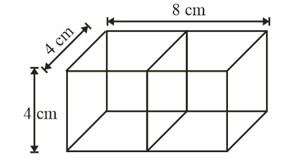#### Two identical cubes each of volume 64 cm3 are joined together end to end. What is the surface area of the resulting cuboid?

It is given that volume of cube = 64 cm3

( Because the volume of cube = a3 )

So the side of the two cubes are 4 cm

The cuboid formed by joining two cubes.The surface area of the cuboid